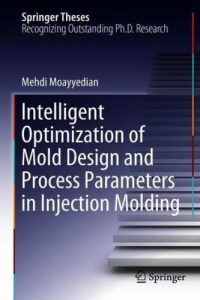اسم المؤلف
Mehdi Moayyedian
التاريخ
8 أكتوبر 2019
المشاهدات
136
التقييم(لا توجد تقييمات)Loading...

Intelligent Optimization of Mold Design and Process Parameters in Injection Molding
Mehdi Moayyedian
Doctoral Thesis accepted by University of South Australia, Adelaide, Australia
xi Value at different levels
nAi Number of levels
Ai Value at level i of factor A
MSA Mean square of factor A
MST Total mean square
MSE Mean square of error variance
FA Value of the F ratio of factor A
PC Percentage of contribution
A Gate type
B Filling time
C Part cooling time
D Pressure holding time
E Melt temperature
a Positive factor
b Negative factor
k Regular factor
SjðXjÞ P-dimensional variable weight profit vector
W Variable weight vector
s Variable weight state vector
w Constant weight vector
m Trial number of experiment
n Number of injection defects
~vij Normalized positive trial numbers
Aþ Fuzzy positive ideal solution
A Fuzzy negative ideal solution
di The distance of each alternative or experiments
QIi Quality index of the n alternative
a~1 Rating the seriousness (very low)
a~2 Rating the seriousness (low)
a~3 Rating the seriousness (medium)
a~4 Rating the seriousness (high)
a~5 Rating the seriousness (very high)
R~ Fuzzy relative matrix
V~ Varied weight fuzzy evaluation matrix
W~ Constant weight factor matrix
MIi Moldability index
D0 Diameter of injected parts before shrinkage
D00 Diameter of injected parts after shrinkage
KA Number of level for factor A
كلمة سر فك الضغط : books-world.net